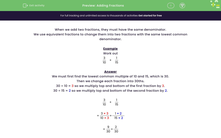### Comprehensive & curriculum aligned

In this worksheet, students will add two simple fractions by first finding their lowest common denominator.Key stage:  KS 3

Curriculum topic:   Number

Curriculum subtopic:   Use Four Operations for All Numbers

Popular topics:   Fractions worksheets, Percentages worksheets

Difficulty level:#### Worksheet Overview

When we add two fractions, they must have the same denominator.

We use equivalent fractions to change them into two fractions with the same lowest common denominator.

Example

Work out

 3 + 1 10 15

We must first find the lowest common multiple of 10 and 15, which is 30.

Then we change each fraction into 30ths.

30 ÷ 10 = 3 so we multiply top and bottom of the first fraction by 3.

30 ÷ 15 = 2 so we multiply top and bottom of the second fraction by 2.

 3 + 1 10 15

 = 3 × 3 + 1 × 2 10 × 3 15 × 2

 = 9 + 2 30 30

 = 11 30

We must always simplify or reduce our answer to its lowest possible terms.

### What is EdPlace?

We're your National Curriculum aligned online education content provider helping each child succeed in English, maths and science from year 1 to GCSE. With an EdPlace account you’ll be able to track and measure progress, helping each child achieve their best. We build confidence and attainment by personalising each child’s learning at a level that suits them.

Get started#### Popular Maths topics

••••